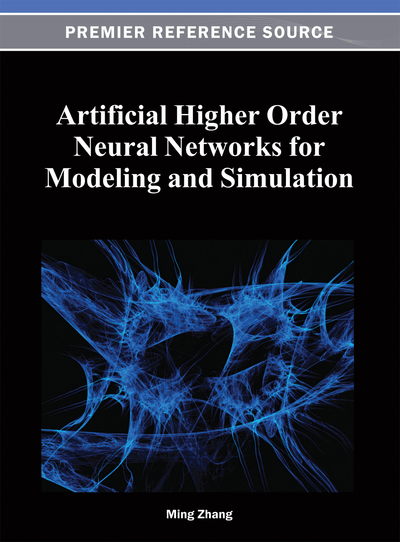# Artificial Polynomial and Trigonometric Higher Order Neural Network Group Models

Ming Zhang (Christopher Newport University, USA)
DOI: 10.4018/978-1-4666-2175-6.ch005
Available
\$37.50
No Current Special Offers

## Abstract

Real world financial data is often discontinuous and non-smooth. Accuracy will be a problem, if we attempt to use neural networks to simulate such functions. Neural network group models can perform this function with more accuracy. Both Polynomial Higher Order Neural Network Group (PHONNG) and Trigonometric polynomial Higher Order Neural Network Group (THONNG) models are studied in this chapter. These PHONNG and THONNG models are open box, convergent models capable of approximating any kind of piecewise continuous function to any degree of accuracy. Moreover, they are capable of handling higher frequency, higher order nonlinear, and discontinuous data. Results obtained using Polynomial Higher Order Neural Network Group and Trigonometric polynomial Higher Order Neural Network Group financial simulators are presented, which confirm that PHONNG and THONNG group models converge without difficulty, and are considerably more accurate (0.7542% - 1.0715%) than neural network models such as using Polynomial Higher Order Neural Network (PHONN) and Trigonometric polynomial Higher Order Neural Network (THONN) models.
Chapter Preview
Top

## Introduction

### HONN Models for Simulation

Artificial Higher Order Neural Network (HONN) is powerful technique to simulate data. Ghosh and Shin (1992) develop efficient higher-order neural networks for function approximation and classification. Zhang, Murugesan, and Sadeghi (1995) design polynomial higher order neural network for economic data simulation. Zhang, Fulcher, and Scofield (1996) study neural network group models for estimating rainfall from satellite images. Zhang, Zhang, and Fulcher (1997) explore financial simulation system using a higher order trigonometric polynomial neural network group model. Lu, Qi, Zhang, and Scofield (2000) provide PT-HONN models for multi-polynomial function simulation. Zhang and Lu (2001) research financial data simulation by using Multi-Polynomial Higher Order Neural Network (M-PHONN) model. Zhang (2001) deliver a new model, called Adaptive Multi-Polynomial Higher Order Neural Network (A-PHONN), for financial data simulation. Qi and Zhang (2001) test the rainfall estimation using M-PHONN model and find that the M-PHONN model for estimating heavy convective rainfall from satellite data has 5% to 15% more accuracy than the polynomial higher order neural network. Zhang and Scofield (2001) expand an adaptive multi-polynomial high order neural network (A-PHONN) model for heavy convective rainfall estimation and find that the A-PHONN model has 6% to 16% more accuracy than the polynomial higher order neural network. Zhang (2003) intends PL-HONN model for financial data simulation. Rovithakis, Chalkiadakis, and Zervakis (2004) learn the high-order neural network structure selection for function approximation applications using genetic algorithms. Crane and Zhang (2005) discover the data simulation using SINCHONN Model. Zhang (2005) gives a data simulation system using sinx/x and sin x polynomial higher order neural networks. Zhang (2006) seeks a data simulation system using CSINC polynomial higher order neural networks. Zhang (2009a) delivers general format of Higher Order Neural Networks (HONNs) for nonlinear data analysis and six different HONN models. This chapter mathematically proves that HONN models could converge and have mean squared errors close to zero. This chapter illustrates the learning algorithm with update formulas. HONN models are compared with SAS Nonlinear (NLIN) models and results show that HONN models are 3 to 12% better than SAS Nonlinear models. Zhang (2009b) develops a new nonlinear model, Ultra high frequency Trigonometric Higher Order Neural Networks (UTHONN), for time series data analysis. Results show that UTHONN models are 3 to 12% better than Equilibrium Real Exchange Rates (ERER) model, and 4 – 9% better than other Polynomial Higher Order Neural Network (PHONN) and Trigonometric Higher Order Neural Network (THONN) models. This study also uses UTHONN models to simulate foreign exchange rates and consumer price index with error approaching 0.0000%. Murata (2010) finds that a Pi-Sigma higher order neural network (Pi-Sigma HONN) is a type of higher order neural network, where, as its name implies, weighted sums of inputs are calculated first and then the sums are multiplied by each other to produce higher order terms that constitute the network outputs. This type of higher order neural networks has accurate function approximation capabilities.

## Complete Chapter List

Search this Book:
Reset